# FRETBursts - 8-spot smFRET burst analysis¶

This notebook is part of a tutorial series for the FRETBursts burst analysis software.

For a step-by-step introduction to FRETBursts usage please refer to us-ALEX smFRET burst analysis.

In this notebook we present a typical FRETBursts workflow for multi-spot smFRET burst analysis. Briefly, we show how to perform background estimation, burst search, burst selection, FRET histograms, and FRET efficiency fit using different methods.

In :
from fretbursts import *

 - Optimized (cython) burst search loaded.
- Optimized (cython) photon counting loaded.
--------------------------------------------------------------
You are running FRETBursts (version 0.6.4).

If you use this software please cite the following paper:

FRETBursts: An Open Source Toolkit for Analysis of Freely-Diffusing Single-Molecule FRET
Ingargiola et al. (2016). http://dx.doi.org/10.1371/journal.pone.0160716

--------------------------------------------------------------

In :
sns = init_notebook()

In :
import lmfit; lmfit.__version__

Out:
'0.9.7'
In :
import phconvert; phconvert.__version__

Out:
'0.7.3'

Here we download an 8-spot smFRET measurement file using the download_file in FRETBursts:

In :
url = 'http://files.figshare.com/2182604/12d_New_30p_320mW_steer_3.hdf5'

In :
download_file(url, save_dir='./data')

URL:  http://files.figshare.com/2182604/12d_New_30p_320mW_steer_3.hdf5
File: 12d_New_30p_320mW_steer_3.hdf5



# Selecting a data file¶

In :
filename = "./data/12d_New_30p_320mW_steer_3.hdf5"

In :
import os
assert os.path.exists(filename)


In :
d = loader.photon_hdf5(filename)


For convenience we can set the correction coefficients right away so that they will be used in the subsequent analysis. The correction coefficients are:

• leakage or bleed-through: leakage
• direct excitation: dir_ex (ALEX-only)
• gamma-factor gamma

The direct excitation cannot be applied to non-ALEX (single-laser) smFRET measurements (like the current one).

In :
d.leakage = 0.038
d.gamma = 0.43


NOTE: at any later moment, after burst search, a simple reassignment of these coefficient will update the burst data with the new correction values.

Compute background and burst search:

In :
d.calc_bg(bg.exp_fit, time_s=30, tail_min_us='auto', F_bg=1.7)
d.burst_search(L=10, m=10, F=7)

 - Calculating BG rates ... [DONE]
- Performing burst search (verbose=False) ...[DONE]
- Calculating burst periods ...[DONE]
- Counting D and A ph and calculating FRET ...
- Applying background correction.
- Applying leakage correction.
[DONE Counting D/A]


Perform a background plot as a function of the channel:

In :
mch_plot_bg(d)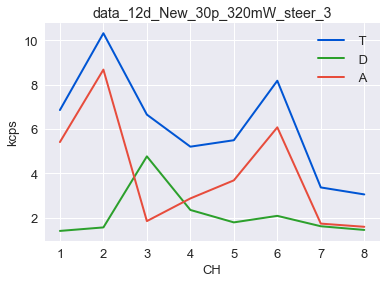Let's take a look at the photon waiting times histograms and at the fitted background rates:

In :
dplot(d, hist_bg);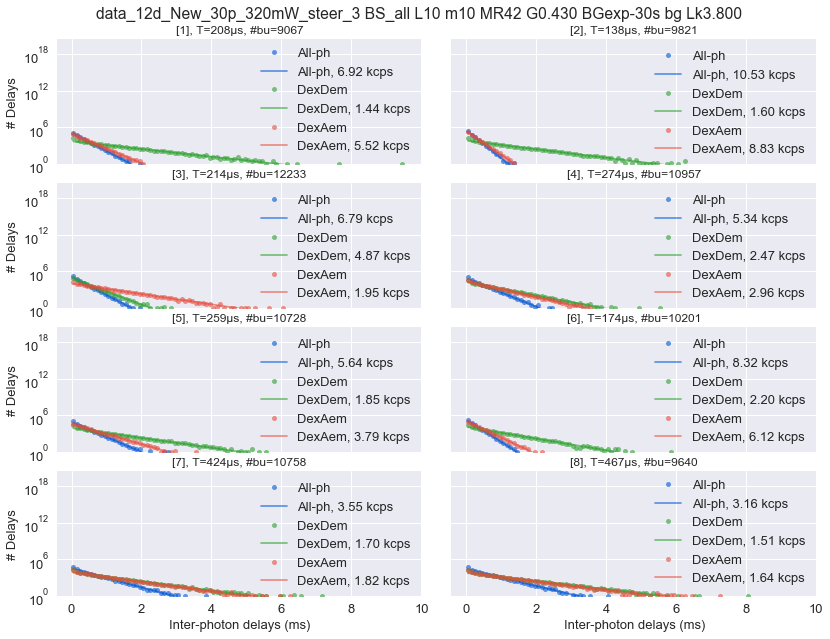Using dplot exactly in the same way as for the single-spot data has now generated 8 subplots, one for each channel.

Let's plot a timetrace for the background to see is there are significant variations during the measurement:

In :
dplot(d, timetrace_bg);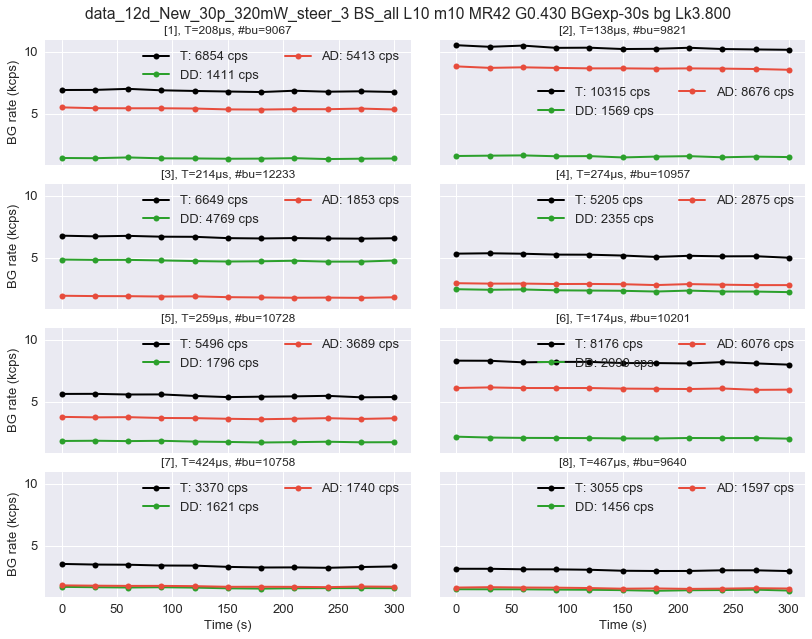We can look at the timetrace of the photon stream (binning):

In :
dplot(d, timetrace)
xlim(2, 3); ylim(-100, 100);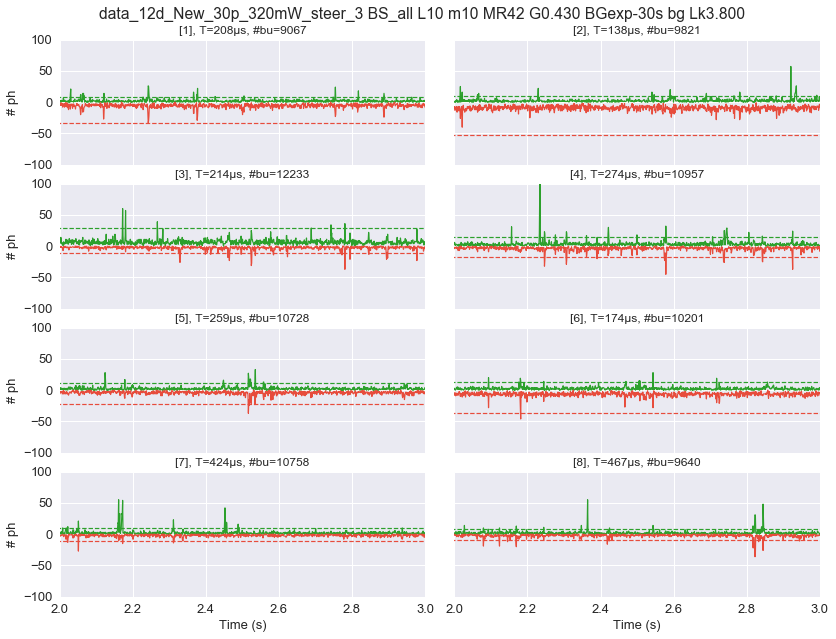We can also open the same plot in an interactive window that allows scrolling (uncomment the following lines):

In :
#%matplotlib qt

In :
#dplot(d, timetrace, scroll=True);

In :
#ylim(-100, 100)

In :
#%matplotlib inline


## Burst selection and FRET¶

### Selecting bursts by burst size (select_bursts.size)¶

In :
gamma = d.gamma
gamma

Out:
array(0.43)
In :
d.gamma = 1
ds = d.select_bursts(select_bursts.size, th1=30, gamma=1)
dplot(ds, hist_fret);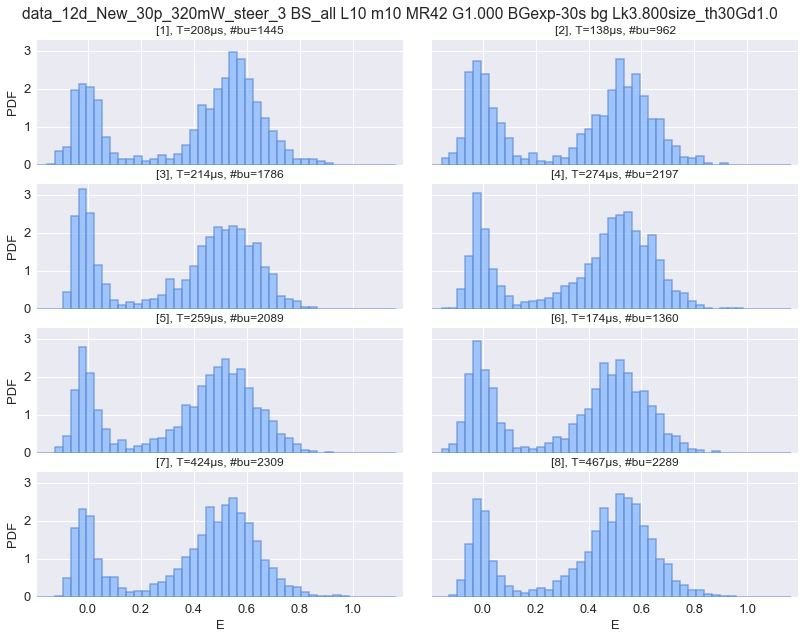In :
ds = d.select_bursts(select_bursts.size, th1=25, gamma=gamma, donor_ref=False)
dplot(ds, hist_fret);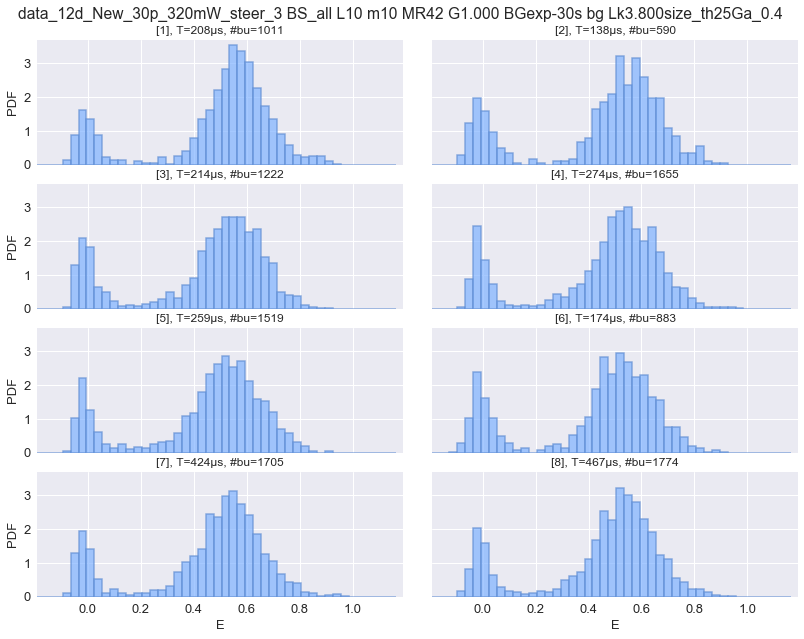In :
ds = d.select_bursts(select_bursts.size, th1=25, gamma=gamma)
dplot(ds, hist_fret, weights='size', gamma=gamma);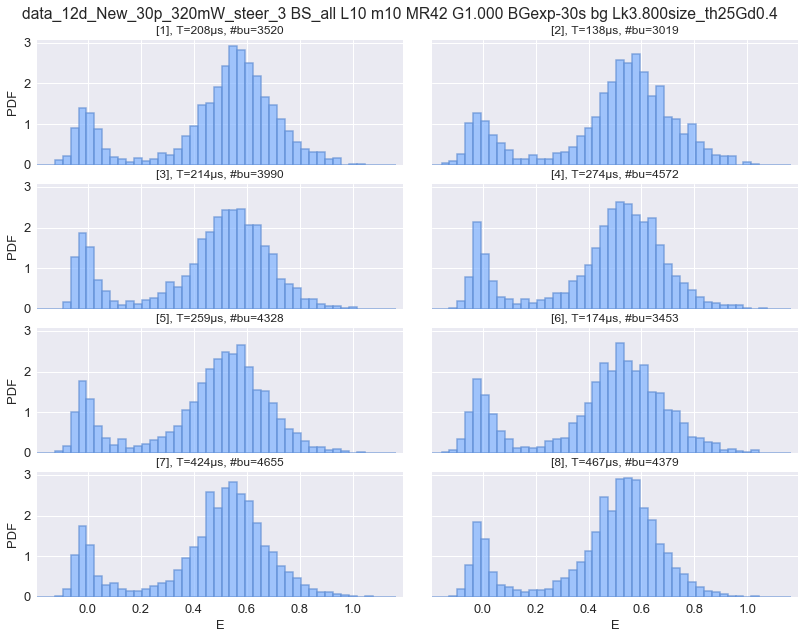In :
dplot(ds, scatter_fret_nd_na); ylim(0,200);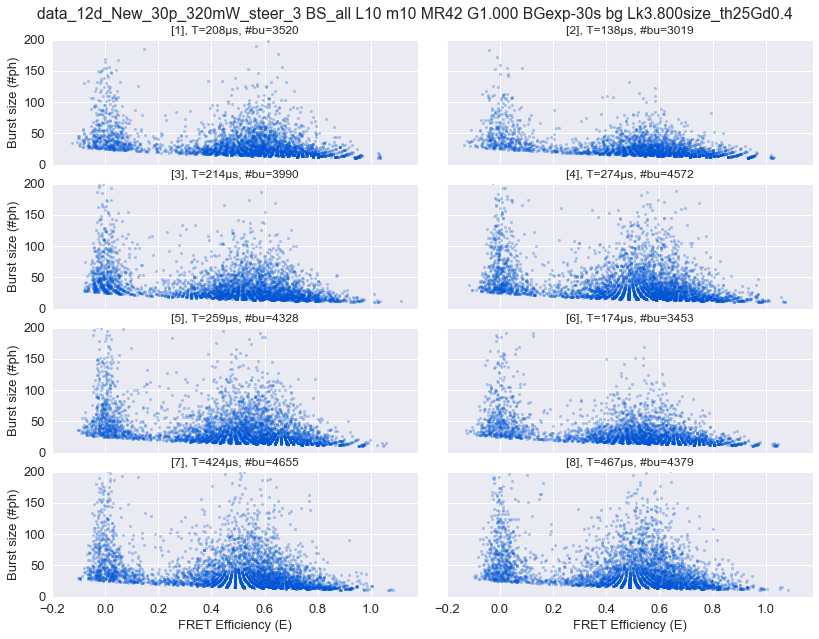## FRET Fitting¶

### 2-Gaussian mixture¶

Let's fit the $E$ histogram with a 2-Gaussians model:

In :
ds.gamma = 1.
bext.bursts_fitter(ds, weights=None)
ds.E_fitter.fit_histogram(mfit.factory_two_gaussians(), verbose=False)


The fitted parameters are stored in a pandas DataFrame:

In :
ds.E_fitter.params

Out:
p1_amplitude p1_center p1_sigma p2_amplitude p2_center p2_sigma
0 0.161037 0.0147363 0.054972 0.827205 0.587919 0.144063
1 0.154906 0.0165125 0.065014 0.830402 0.592552 0.147132
2 0.172021 0.00363337 0.0429549 0.818251 0.56242 0.148298
3 0.151185 0.00576416 0.0410726 0.827219 0.565177 0.14754
4 0.155672 0.00718107 0.0442892 0.830064 0.558945 0.15308
5 0.169139 0.00913894 0.0535911 0.822227 0.561622 0.153229
6 0.15287 0.00624211 0.0480221 0.82579 0.559307 0.143679
7 0.154317 0.00772731 0.0412279 0.822823 0.556762 0.138972
In :
dplot(ds, hist_fret, weights=None, show_model=True,
show_fit_stats=True, fit_from='p2_center');